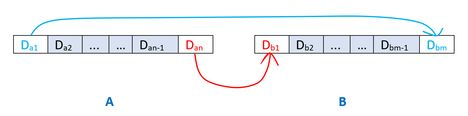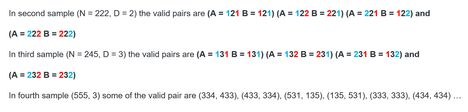# Practice on Toph

Participate in exhilarating programming contests, solve unique algorithm and data structure challenges and be a part of an awesome community.

# Reverse Number

By ashikurrahman · Limits 5s, 512 MB

You are given an integer $N$ and a digit $D$. You have to count the number of pairs $A$ and $B$ ${(1 \le A, B \le N)}$ such that

1. $A$ and $B$ do not contain any leading zeros

2. Last digit of $A$ is equal to the first digit of $B$

3. First digit of $A$ is equal to last digit of $B$ and

4. Except for the the first and last digits of both integer $A$ and $B$, there must be at least one digit present in both $A$ and $B$ which is equal to the given digit $D$.Print the answer modulo $1000000007$$(10^{9} + 7)$.

## Input

First line contains an integer $T$ ${(T \le 100000)}$ denoting test cases. Then $T$ line follows two space separated integers $N$ and $D$.

$1 <= N <= 10^{18}$

$0 <= D <= 9$

## Output

Print a single line the the number of pair satisfy the condition for each test cases.

## Sample

InputOutput
5
111 1
222 2
245 3
555 3
100 5

1
4
4
25
0### Statistics

50% Solution Ratio

kzvd4729Earliest, 6M ago

YouKnowWhoFastest, 1.2s

kzvd4729Lightest, 1.0 MB

Deshi_TouristShortest, 1532B

### Submit